## Selina Concise Mathematics Class 10 ICSE Solutions Chapter 9 Matrices Ex 9B

These Solutions are part of Selina Concise Mathematics Class 10 ICSE Solutions. Here we have given Selina Concise Mathematics Class 10 ICSE Solutions Chapter 9 Matrices Ex 9B.

Other Exercises

Question 1.
Evaluate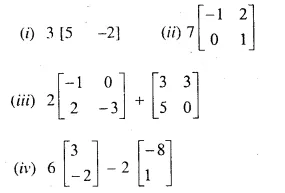Solution: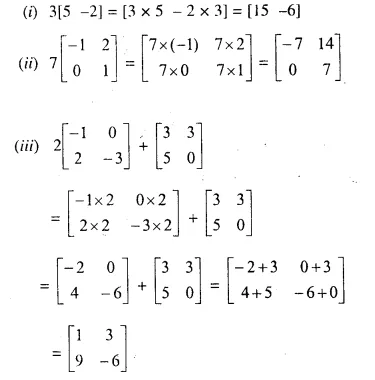Question 2.
Find x and y if :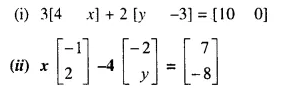Solution: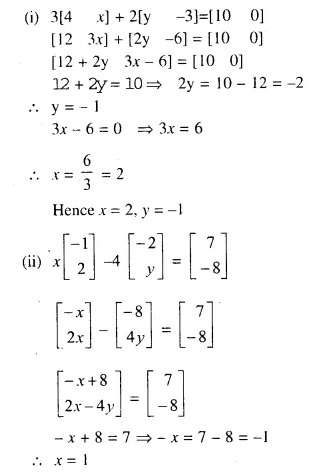Question 3.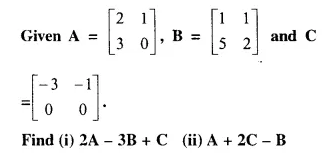Solution: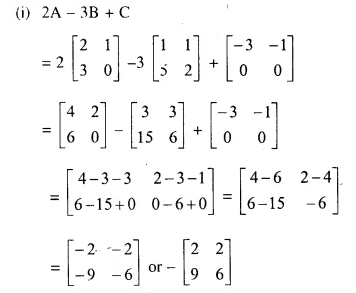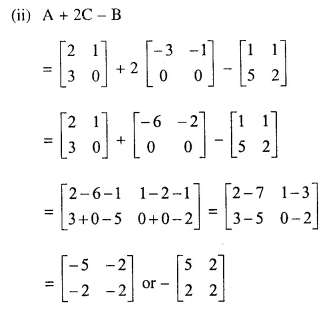Question 4.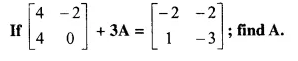Solution: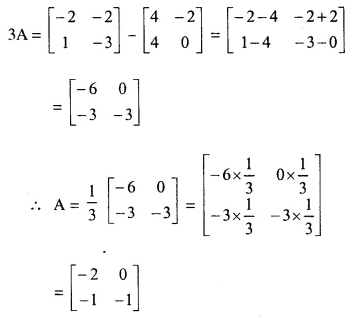Question 5.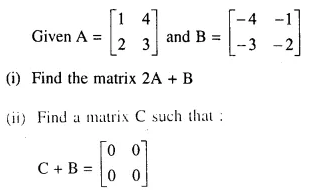Solution:Question 6.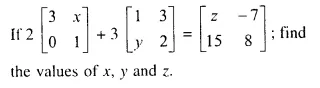Solution: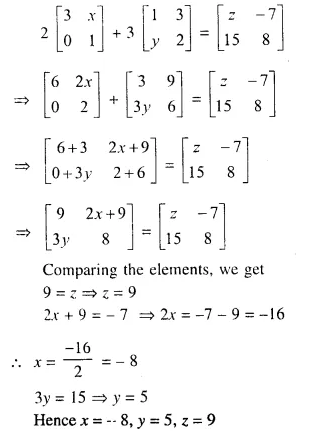Question 7.Solution: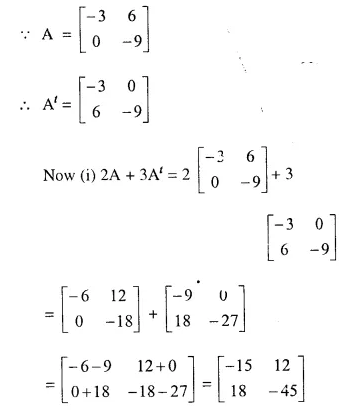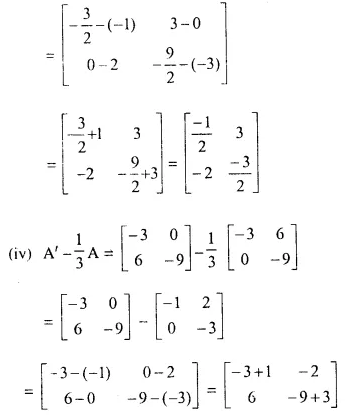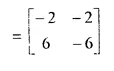Question 8.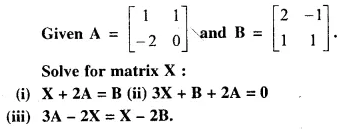Solution: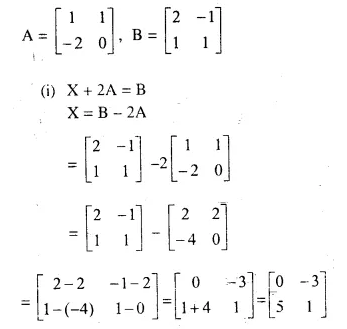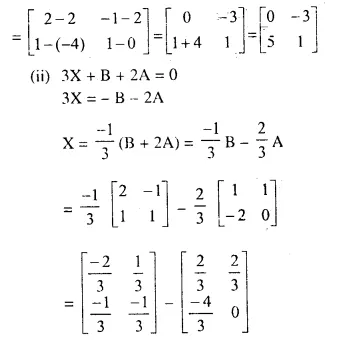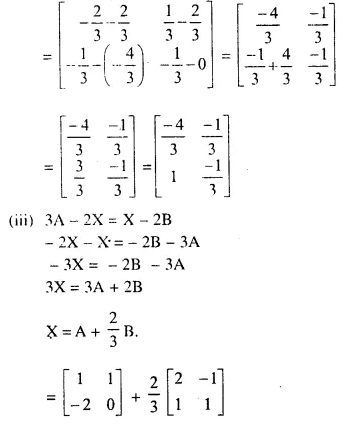Question 9.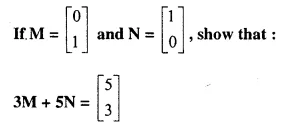Solution: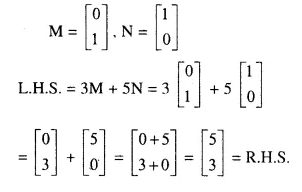Question 10.
If I is the unit matrix of order 2 x 2; find the matrix M, such that: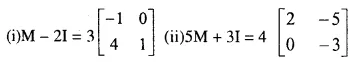Solution: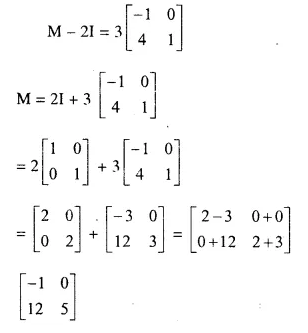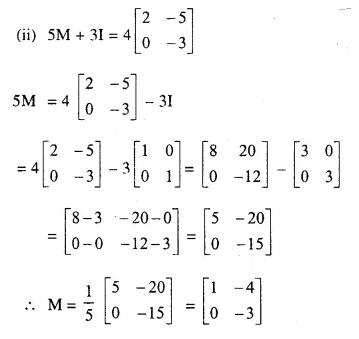Question 11.Solution: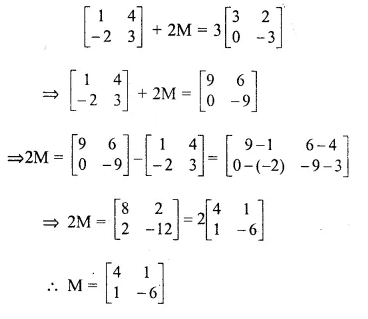Hope given Selina Concise Mathematics Class 10 ICSE Solutions Chapter 9 Matrices Ex 9B are helpful to complete your math homework.

If you have any doubts, please comment below. Learn Insta try to provide online math tutoring for you.